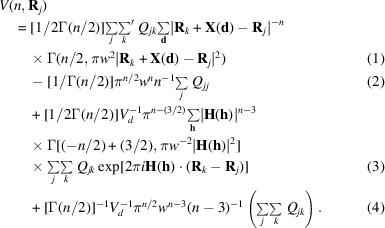International
Tables for
Crystallography
Volume B
Reciprocal space
Edited by U. Shmueli

International Tables for Crystallography (2006). Vol. B, ch. 3.4, pp. 388-389   | 1 | 2 |

Section 3.4.5. Extension of the method to a composite lattice

D. E. Williamsa

aDepartment of Chemistry, University of Louisville, Louisville, Kentucky 40292, USA

3.4.5. Extension of the method to a composite lattice

| top | pdf |

Define a general lattice sum over direct-space points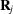which interact with pairwise coefficients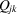, where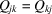: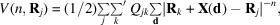where the prime indicates that when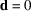the self-terms withare omitted. For convenience the terms may be divided into three groups: the first group of terms has, where j is unequal to k; the second group has d not zero and j not equal to k; and the third group had d not zero and. (A possible fourth group withandis omitted, as defined.)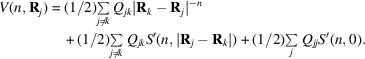By expanding this expression we obtain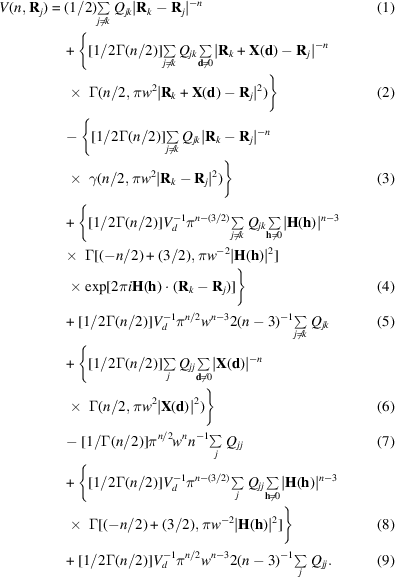This expression for V has nine terms, which are numbered on the right-hand side. Term (3) can be expressed in terms of Γ rather than γ: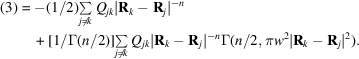It is seen that cancellation occurs with term (1) so that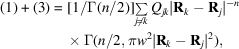which is the, j unequal to k portion of the treated direct-lattice sum. The d unequal to 0, j unequal to k portion corresponds to term (2) and the d unequal to 0,portion corresponds to term (6). The direct-lattice terms may be consolidated as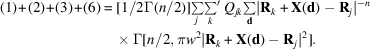Now let us combine terms (4) and (8), carrying out the h summation first: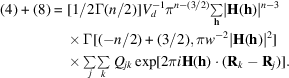Terms (5) and (9) may be combined: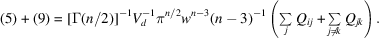The final formula is shown below. The significance of the four terms is: (1) the treated direct-lattice sum; (2) a correction for the difference resulting from the removal of the origin term in direct space; (3) the reciprocal-lattice sum, except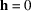; and (4) theterm of the reciprocal-lattice sum.